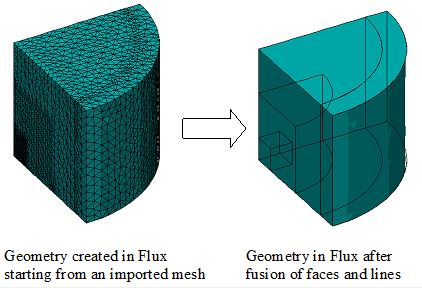# Stage of fusion

## Introduction

Following the import, the geometry of the imported device has multiple lines and faces deriving from multiple facets and edges of the initial file.

The second stage is the stage of fusion (regrouping of the entities), which enables the reduction of number of lines and faces, and facilitates their handling, as well as the visualization of the device.

## Fusion of faces: use

Although strongly advised, the fusion of faces / lines is optional. This operation becomes compulsory for the faces in the cases presented below:

If … The fusion …
kinematic coupling of dissociation faces (sliding cylinder, boundary of mobile mechanical set and compressible mechanical set)
symmetry and/or periodicity planes of faces located on these planes
… is compulsory

## Concept of fusion

We call fusion of faces / lines the operation of regrouping faces / lines to form the main faces / lines of the device geometry.

## Principle of fusion of faces and data structure

The principle of fusion of faces is shown on the scheme below. During fusion all faces belonging to the same surface are regrouped in one face.The faces resulting from mesh import are faces defined by a list of facets.

• Before the fusion of faces:

every face (of facets list type) contains a single facet

• After the fusion of faces:

every face (of facets list type) contains many facets

## Regrouping surface and angle of fusion

The surface of regrouping is defined by the user, using an angle named angle of fusion. All adjacent faces whose angle is less than the fusion angle are regrouped in a single face (See figure of example below).

Example: Three adjacent faces are regrouped in a single face with a fusion angle α:The regrouping surfaces can be of different shapes (plane, cylindrical, …) and depend on the chosen value of fusion angle as follows:

• for an angle of small value (between 0 and 1°), the regrouping surface is a planar surface
• for a larger angle, the regrouping surface can be of any shape

## Precaution

So that the simplified geometry approaches with more real geometry, it is necessary to take some care as for the choices of an angle of fusion, the risk being to gather faces, which should remain separate.

In general, it is advised to comply with the following rule:

• start with an angle that is inferior or equal to 1° to identify the plane faces
• gradually increase the value of the angle to identify the other faces

## Attention

The fusion process does not create even surfaces. The regrouping surface is an uneven surface (although this surface looks like an even one).

## And for the lines …

The principle of lines fusion is the same with the one of faces fusion. It is illustrated in the figure below.## Rules of fusion

Two faces (lines) can be regrouped if they belong to same volumes (faces).

The mesh import of a quarter cylinder before and after the fusion of faces and lines is shown in the figure below.